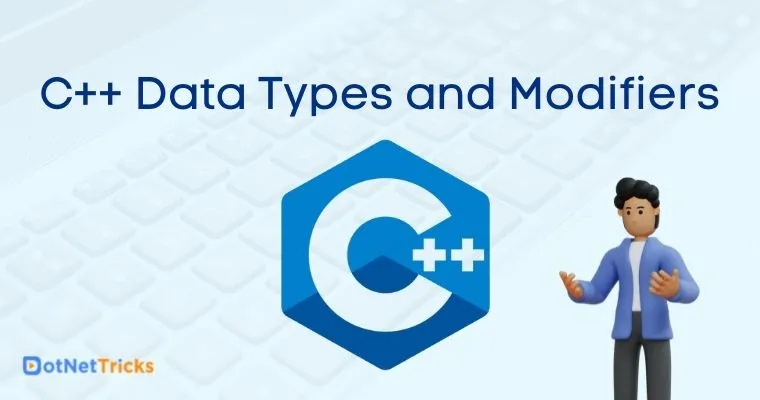# C++ Data Types and Modifiers

22 Aug 2022
Beginner
5.57K Views

Hello readers. This article will cover the basics of data type in C++ language. If you want to get started with the basics of C++, its history and write basic programs, then please refer this Getting Started with C++ Language article.

## Data type in C++

When writing a program, variables are used to store some information. It’s important to decide which variable stores what kind of information. Data type helps the compiler to understand what type of data can be stored into a particular variable.

For e.g., variable ‘a’ can hold only integer values, while ‘b’ can hold only character values. It is very important that you append the appropriate data type for the variable. Irrespective of defining a variable or not, the compiler automatically allocates memory space for the data type.

## Types of Data types in C++

The data types in C++ are divided into two different types –

1. Primitive Data types

2. Abstract Data types

This section will explain the different data types in detail.

## Primitive Data types

Primitive data type is also referred to as “Built-in data type”. Primitive data types are pre-defined, and they can be used when writing programs to define variables. Primitive data types are not composed of any other data types. The list of primitive data types are:

• Integer

• Character

• Float

• Double

• Void

### Integer Data type

Integer data type comprises of numbers without the decimal (fraction) part. Example: 34, 999, -5287, etc. Integer data types can be positive and/or negative values.

• Keyword used to define an integer data type : int

• Number of bytes of memory space required : 4 bytes

• Range of integer data type : -2147483648 to 2147483647

#### Example Program

In this program, we will store 2 integer values in variables a and b, perform a sum operation of the two integer values and store the result in a third variable (result). We will print the value stored in the result variable.

```#include <iostream>
using namespace std;

int main ()
{
int a, b;
int result;
a = 3;
b = 4;
result = a + b;
cout << result;
return 0;
}
```

The output of this program will be: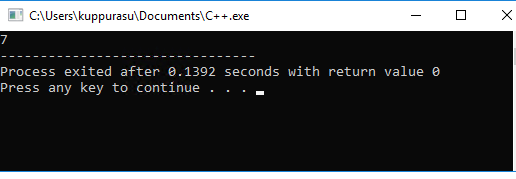### Character Data type

Character data type has the capability to store any single character from the ASCII character set. It can be an alphabet, a digit, space or punctuation. The characters are internally stored as integers between the range of -128 to 127. Therefore, the character data type can also be treated as an integer data type.

• Keyword used to define an integer data type : char

• Number of bytes of memory space required : 1 byte (only one character at a time)

• Range of integer data type: -128 to 127

#### Example Program

In this program, we will ask the user to enter a character. We will store the character in a variable (a) defined as a character data type. We will then print the value stored in the character data type variable.

```#include<iostream>
using namespace std;

int main ()
{
char a;
cout<< "Enter a character: ";
cin >:> a;
cout << "The character you entered is: "<< a;
return 0;
}
```

The output of this program will be: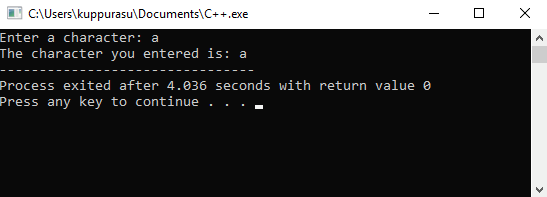### Float Date type

Float data type refers to any integer having a decimal part. Example: 15.6744, 763.09124, etc. are referred to as floating point numbers. If you write the number 25, it is an integer. While 25.0 is a floating-point number. You must use the floating-point data type when you make some calculations such as average, percentage and so on.

• Keyword used to define an integer data type : float

• Number of bytes of memory space required : 4 bytes

• Range of integer data type : +/- 3.4e +/- 38 (~7 digits precision)

#### Example Program

In this program, we will perform a mathematical calculation to convert miles to kilometers. We will collect the miles as user input and store it in a variable. We will multiply the user input with a value of 1.60934 and store the result in another variable (km). Finally, we will print the value of the (km) variable to the user.

```#include <iostream>
using namespace std;
int main ()
{
float m;
float km;
cout << "Enter the distance in miles:";
cin >> m;
km = m * 1.60934;
cout << "The distance in kilometer is: "<< km;
}
```

The output of this program will be :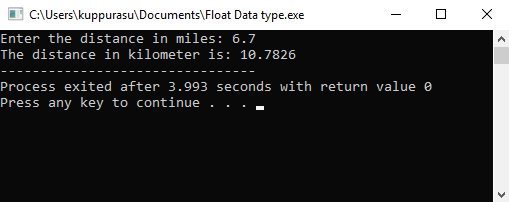### Double Data type

Double data type can be used to handle floating point values. But the difference from the float data type is that double data type occupies double the memory size. Double data type can store more numbers after the decimal point (~15 digits).

• Keyword used to define an integer data type : double

• Number of bytes of memory space required : 8 bytes

• Range of integer data type : +/- 1.7e to +/- 308 (~15 digits precision)

#### Example Program

```
#include <iostream>
using namespace std;
int main ()
{
double a = 5426904022.9684902434392323;
cout << "Double Floating Point:"<< a;
}
```

The output of this program will be –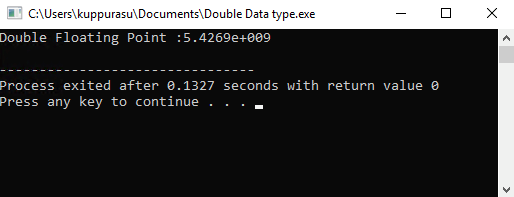### Void Data type

Void data type can be used to specify an empty parameter list for a function as well as a return type for the function. When void is specified, no memory will be allocated. When it is used to specify an empty parameter list, it means that the function will not take any arguments. When used as a return type for the function, the function will not return any value.

## Abstract Data types

Abstract data types or derived data types are data types that are derived from primitive data types using specific declaration operators. The abstract data types are as follows:

• Arrays

• Functions

• Pointers

• References

• Class

• Structures

## Datatype Modifiers

In the previous section, we discussed about the data types in C++. In this section, we will take a look at the modifiers that will precede the data type. Other than the void data type, all basic data types will have data type modifiers. The types of modifiers are

• Signed

• Unsigned

• Long

• Short

Let’s take a look at how the data type modifiers impact the data types in the below table

Data Type
Data type Modifier
Data type Size
Range
Integer (int)
int
4 bytes
-2147483648 to 2147483647
Unsigned int
4 bytes
0 to 4294967295
Signed int
4 bytes
-2147483648 to 2147483647
Short int
2 bytes
-32768 to 32767
Unsigned short int
Range
0 to 65,535
Signed short int
Range
-32768 to 32767
Long int
4 bytes
-2,147,483,648 to 2,147,483,647
Signed Long int
4 bytes
-2,147,483,648 to 2,147,483,647
Unsigned Long int
4 bytes
0 to 4,294,967,295
Char
Signed Char
1 byte
-127 to 127
Unsigned Char
1 byte
0 to 255
Float
Float
4 bytes
+/- 3.4e +/- 38 (~7 digits
Double
Double
8 bytes
+/- 1.7e +/- 308 (~15 digits)
Long Double
8 bytes
+/- 1.7e +/- 308 (~15 digits)
##### Summary

In this section, we have covered the following topics:

• What is a data type in C++

• Types of data types

• Primitive data type - (integer, character, float, double, void)

• Abstract data type - (array, function, pointers, references, class, structure)

• Data type modifiers in C++

In the next section, we will take a look at the different variables that can be defined with these data types in C++.

Learn to Crack Your Technical Interview